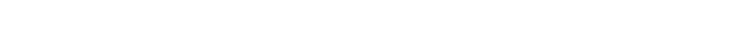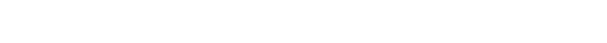# Powers of the golden ratio

My interest in continued fractions was sparked by working out how to knit the stars in the EU flag earlier this year. It turned out that 13/15 was a remarkably good approximation for √3/2, which was crucial for placing the stars.

While exploring the world of continued fractions, I came across a reference to a seemingly unrelated fact: the powers of the golden ratio (1/2 + √5/2) are remarkably close to integers. The effect starts to show up from the 4th power and becomes very obvious thereafter:

(1/2 + √5/2)4  =    6.85

(1/2 + √5/2)5  =  11.09

(1/2 + √5/2)6  =  17.94

(1/2 + √5/2)7  =  29.03

(1/2 + √5/2)8  =  46.98

As John D Cook shows in his blog, the values become closer to integers at an exponential rate as the powers increase. What was more difficult to find was any clear explanation of why the powers approach integers. The link between the golden ratio and the Fibonacci numbers, however, provides an intriguing clue.

First, it’s worth noting that the integers to which the powers above converge are sums of two Fibonaccci numbers. Let’s start by labelling numbers in the Fibonacci sequence as follows:Comparing this with the sequence of powers above, it’s apparent that

(1/2 + √5/2)5  ≈  F4 + F6

(1/2 + √5/2)6  ≈  F5 + F7

and in general we can say that (1/2 + √5/2)n ≈ Fn-1 + Fn+1.

Second, if we expand the powers of the golden ratio, Fibonacci numbers appear again:In fact the Fibonacci numbers appear in two ways. If we look (left side of table) at the coefficient of the √5 term for say the 4th power, we see it’s 3/2 – or half of F4. More generally, the coefficient of the √5 term for the nth power is half of the nth Fibonacci number.

But we can alternatively express the powers in terms of the golden ratio, as on the right-hand side of the table. This is in itself a remarkable property, and is a convenient way of looking at the power series. Note that each power can be expressed as a Fibonacci multiple of the GR plus another Fibonacci number. For example, the 4th power is F4 x GR + F3. And more generally, the nth power is Fn x GR + Fn-1.

The final piece of the jigsaw involves the continued fraction for the golden ratio. This is one of the simpler continued fractions, being made up entirely of 1s:It produces approximations (or convergents) made up – once again – of Fibonacci numbers:The first few approximations are grossly inaccurate, but by the time we get to A5 the approximation is accurate to around 1%, and A8 is within 0.05%. Note also that, in general, the nth approximation is Fn+1/Fn.

This means that, for example, if we select the 4th approximation and substitute it for the golden ratio in the expression for the 4th power (on the right of the table above), the denominator of the approximation cancels out to produce an integer.This example comes early in the sequence, and 7 is not a particularly good approximation for GR4. But as we go up the sequence of powers, substituting the nth approximation for the GR in the expression for the nth power, the denominators cancel out each time and we get better and better approximations.I would hesitate to say that this “explains” the phenomenon of near-integer powers – there’s no doubt some deeper, underlying reason why the sequence of approximations fits the sequence of powers so neatly. But looking at the powers in this way does help to make some sense of what’s happening as the powers approach integers.

#### Is this peculiar to the golden ratio?

Much has been written – including much nonsense – about the special properties of the golden ratio. But this isn’t just a peculiar feature of that number.

My internet surfing landed on a reference to a similar property for √2 + 1 (sometimes known as the silver ratio), whose powers converge towards integers even more quickly:

(1 + √2)3  =  14.07

(1 + √2)4  =  33.97

(1 + √2)5  =  82.01

(1 + √2)6  =  197.99

(1 + √2)7  =  478.002

(1 + √2)8  =  1153.999

If we expand the sequence of powers, we can compare this with a sequence of approximations – this time from the continued fraction for √2. Again we see that the two sequences match up, so that the denominator of the approximation cancels out to produce an integer:Beyond 1 + √2 and the golden ratio, we can easily find other numbers with the same property. As a starting-point, note that 1 + √2 is is a root of the equation x2 – 2x = 1, while the golden ratio is the root of the equation x2 – x = 1.

If we look at similar positive roots of equations with the form x2 – nx = 1, a similar phenomenon unfolds. Take for example 2 + √5, which is a root of x2 – 4x = 1. It has powers that converge rapidly towards integers, e.g. (2 + √5)4 = 321.997, and its power sequence fits with the sequence of approximations from the continued fraction for √5:#### A Pisot mystery

In all of these cases, the sequences “zip” together beautifully. A good way to appreciate the orderliness here is by contrast: try out a similar process with numbers such as 1 + √5 or 4 + √5, whose powers don’t converge towards integers, and observe the mess that emerges …

So what do numbers like 1 + √2 and 2 + √5 have in common that 1 + √5 or 4 + √5 don’t? The answer is that they are Pisot numbers (or Pisot-Vijayaraghavan/PV numbers). The class of Pisot numbers includes the positive roots of equations of the form x2 – nx = 1, but is far more extensive.

The quick definition of a Pisot number is that its “conjugate” – with a negative value for the square-root term – lies between -1 and +1. So, taking the examples above, the conjugate of 1 + √2  is 1 – √2, whose value is  -0.414 and hence within the range -1 to +1. The same is true of the conjugate of 2 + √5, which is 2 – √5 and has a value of -0.236. So both 1 + √2 and 2 + √5 qualify as Pisot numbers.

The conjugate of 1 + √5 is 1 – √5, whose value of -1.236, is below the lower end of the range, and the conjugate of 4 + √5 is 4 – √5, whose value of 1.764 lies above the upper end of the range. So neither 1 +√5 nor 4 +√5 are Pisot numbers.

Why that difference should give rise to the phenomenon I’ve explored here is a mystery I haven’t been able to fathom. The concept of PV numbers plunges us straight into the deep end of abstract mathematics and is way beyond the scope of this blog. But getting to know these beautiful creatures should keep me occupied while the government wrecks the country.

[This blog has been included in the 149th edition of Carnival of Mathematics, a monthy round-up of mathematical blogs organised by The Aperiodical.]

[And some of the observations in the blog have been taken further and made mathematically precise in a blog by Igblan.]